Equations with literal coefficientsCustom SearchEQUATIONS WITH LITERAL COEFFICIENTS As stated earlier, the first letters of the alphabet usually represent known quantities (constants), and the last letters represent unknown quantities (variables). Thus, we usually solve for x, y , or 2. An equation such as     ax - 8 = bx - 5 has letters as coefficients. Equations with literal coefficients are solved in the same way as equations with numerical coefficients, except that when an operation cannot actually be performed, it merely is indicated. In solving for x in the equation     ax - 8 =bx-5 subtract bx from both members and add 8 to both members. The result is     ax - bx = 8 - 5 Since the subtraction on the left side cannot actually be performed, it is indicated. The quantity, a - b, is the coefficient of x when terms are collected. The equation takes the form (a - b) x = 3 Now divide both sides of the equation by a-b. Again the result can be only indicated. The solution of the equation is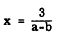In solving for y in the equation ay + b = 4 subtract b from both members as follows: ay = 4 - b Dividing both members by a, the solution isPractice problems. Solve for x in each of the following: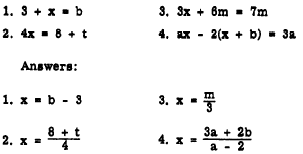REMOVING SIGNS OF GROUPING If signs of grouping appear in an equation they should be removed in the manner indicated in chapter 9 of this course. For example, solve the equation.          5 = 24 - [x-12(x-2) - 6(x-2)] Notice that the same expression, x-2, occurs in both parentheses. By combining the terms containing (x-2), the equation becomes         5 = 24 - [x-18(x-2)] Next, remove the parentheses and then the bracket, obtainingSubtracting 17x from both members and then subtracting 5 from both members, we haveDivide both members by -17. The solution is         x=1 EQUATIONS CONTAINING FRACTIONS To solve for x in an equation such as first clear the equation of fraction, To do this, find the least common denominator of the fraction. Then multiply both rider of the equation by the LCD. The least common denominator of 3, 12, 4, and 2 is 12. Multiply both rider of the equation by 12. The resulting equation is     8x + x - 12 - 3 + 8x Subtract 6x from both members, add 12 to both members, and collect like terms as follows: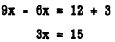The solution is         x= 5 To prove that x = 5 is the correct solution, substitute 5 for x in the original equation and show that both sides of the equation reduce to the same value. The result of substitution is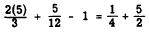In establishing an identity, the two sides of the equality are treated separately, and the op the equality, and it is desirable to find the least common denominator for more than one set of fractions. The same denominator could be used on both sides of the equality, but this might make some of the terms of the fractions larger than necessary. Proceeding in establishing the identity for x = 5 in the foregoing equation we obtain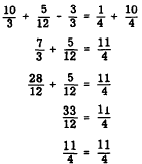Each member of the equality has the value 11/4 when x = 5. The fact that the equation be proves that x = 5 is the solution. Practice problems. Solve each of the following equations: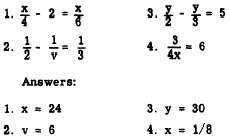Integrated Publishing, Inc. - A (SDVOSB) Service Disabled Veteran Owned Small Business